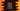# Python program to append a string to a list## Python program to append a string to a list:

In this post, we will learn how to append a string to a list in Python. Sometimes, we need to append a string to a list of strings or to a list of numbers. This can be done easily in Python.

Python provides a couple of easy way to add a string to a list. In this post, we will learn how to do that in different ways with examples.

### Method 1: By using + operator:

This is the easiest way to add any string to a list. But, we can use + with two lists. For that, we need to convert the string to a list before adding it to the original list.

For example, let’s consider the below example:

``````given_list = [1, 2, 3, 4, 5]
given_string = 'hello'

given_list += [given_string]

print(given_list)``````

Here,

• given_list is the given list or original list.
• given_string is the given string.
• We are appending the string to the end of the list by converting it to a list first. The result value is assigned to given_list.
• The last line is printing the value of given_list.

If you run this program, it will print the below output:

``[1, 2, 3, 4, 5, 'hello']``

So, the string is appended to the end of the list.

### Method 2: By using append():

append() is another method we can use to append a string to a list. The below program does the same thing:

``````given_list = [1, 2, 3, 4, 5]
given_string = 'hello'

given_list.append(given_string)

print(given_list)``````

If you run it, it will print the same output.

``[1, 2, 3, 4, 5, 'hello']``